# The Eigenwalker Model of the Human Gait

A model of the human gait, developed by Nikolaus Troje, is a five-term Fourier series with vector-valued coefficients that are the principal components for data obtained in motion capture experiments involving subjects walking on a treadmill.

### Contents

#### Nikolaus Troje

Today's post is about work done several years ago by Nikolaus Troje when he was at Ruhr-Universität in Bochum, Germany. He has since moved to Queens University in Ontario, Canada, where he is head of the Biomotion Lab. Here is their Web page.

Troje studies the human gait. How exactly do we move when we walk? He also studies how we perceive others when they walk. Can we tell if people are happy or sad just by the way they walk? Can we determine if they are male or female?

To get a feeling for his work, take a look at this demo: BMLgender. When I first saw this demo it was being done with MATLAB, so I asked Troje about it and I have written and talked about it many times ever since. (Today, the demo is computed in the web browser with Java Script.)

#### Motion Capture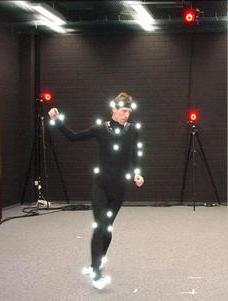Motion capture employs high speed cameras, lights, and reflectors attached to human subjects -- German grad students -- as they walk on a closed track or a treadmill. Once a steady, comfortable pace is established for a single subject, 20 or so steps are recorded. At 120 frames per second, this results in typically 1440 frames. The data from the reflectors are collated into 15 standard locations on the head, arms, torso and legs. As these points move in three dimensions the raw data captured is an array of time series with 45 rows and roughly 1440 columns.

Here are four of the subjects, with the data scaled so that they are all walking at the same speed.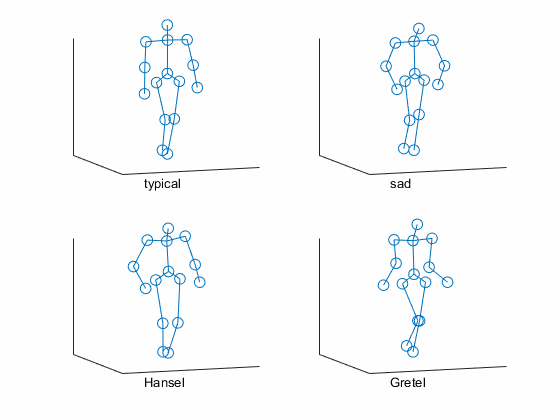#### Fourier and PCA

The walking motion is periodic so Troje's model is a five-term Fourier series describing the position of the 15 spots in three dimensions.

$$p(t) = v_1 + v_2 \cos{\omega t} + v_3 \sin{\omega t} + v_4 \cos{2 \omega t} + v_5 \sin{2 \omega t}$$

Here $\omega$, the scalar frequency, is the only nonlinear parameter. It is determined by Fourier analysis of the data. The coefficients $v_1$ through $v_5$ are vectors with 45 components. Once $\omega$ is computed the $v$'s are obtained by Principal Component Analysis (also known as Singular Value Decomposition) of the data. PCA effectively reduces the 45-by-1440 data matrix to a 45-by-5 coefficient matrix.

The frequency $\omega$ and the coefficient matrix $V$ with columns $v_1, \ldots, v_5$ are computed just once. Then the motion can be computed for any time $t$ with a matrix-vector product.

$$p(t) = V \ [1 \ \cos{\omega t} \ \sin{\omega t} \ \cos{2 \omega t} \ \sin{2 \omega t}]^T$$

This model usually reproduces the raw data with better than 95% accuracy.

#### Eigenwalker

There were several dozen subjects, each with his or her characteristic coefficient matrix $V$. Collect all these $V$'s together into one giant set of coefficients. Then use PCA again to reduce this to one average set of coefficients. You get eigenwalker, the everyman. Or at least the typisch deutsche junge Akademiker.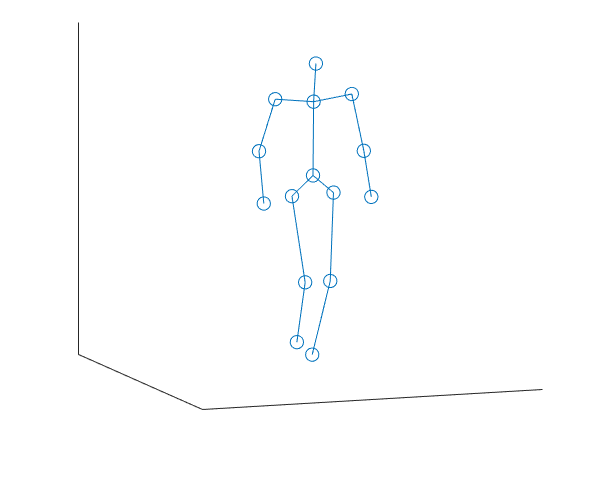#### Principal Components

The eigenwalker has five vector coefficients $v_1, \ldots, v_5$. These components don't have any inherent names -- they're just vectors that come out of the principal component analysis. But when we look at how they affect the motion, we can give them names. The first component, $v_1$, is simply the static position. It determines how eigenwalker looks at rest. Here are the other four components, one at a time.

The second coefficient, $v_2$, dominants the forward motion so we've called it "stride". The third coefficient, $v_3$, controls a sideways motion so it gets named "sway". The coefficients $v_4$ and $v_5$ drive vertical motions that are in-phase and out-of-phase with the stride; they get fairly arbitrary names of "hop" and "bounce".

When these four motions are added to the at-rest position, they give eigenwalker, which is a fairly realistic picture of the human gait.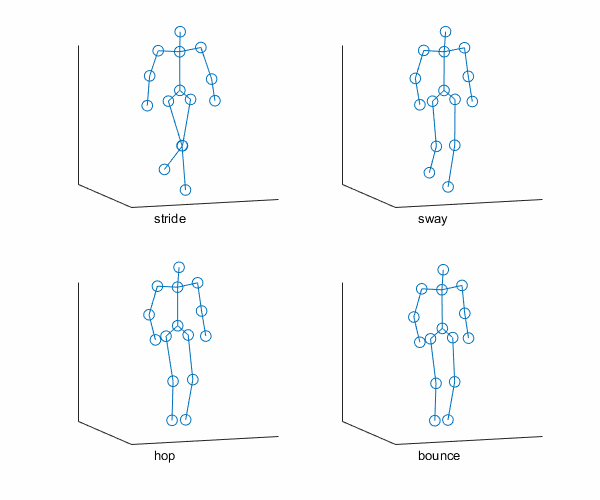#### Gender

The PCA does not pick out gender as one of dominant components. But half of the subjects were female and half were male. So we can compute a matrix of coefficients $F$ for the females and a matrix of coefficients $M$ for the males. The top two figures below are the female eigenwalker and the male eigenwalker. Without looking at the bottom two figures, can you tell which is which?

The bottom two figures overemphasize the gender bias. They run the model with the difference between $F$ and $M$ tripled. Now you can see which is which?

Disney and Pixar know this -- they have to exaggerate the motion to get the point across.

This is Troje's research. How do humans determine the gender of other humans just by watching them walk? Even better -- just by watching a stick figure, or 15 dots, walk?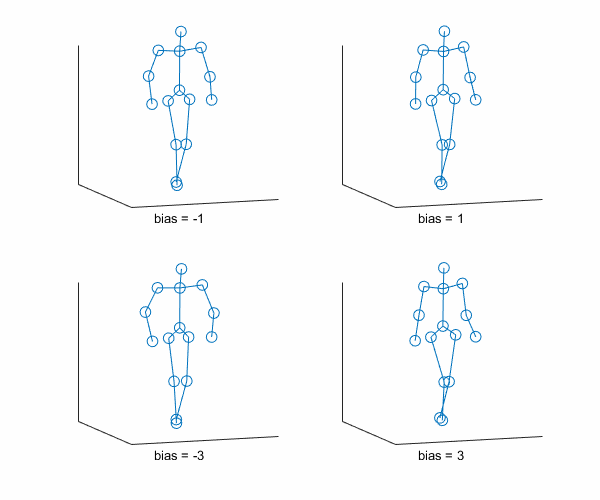#### Eigenwalker App

The Numerical Computing with MATLAB collection of programs includes an app that lets you see the eigenwalker in action. Sliders vary the strength of the five components and the gender bias. You can also rotate the viewpoint. You will need two files, walker.m and walkers.mat, available from the MATLAB Central File Exchange.

#### Disclaimer

This has been my own description of an approach that Troje took over a decade ago. He has since replaced Principle Components Analysis with Fourier analysis to represent the movements of an individual walker, and has applied PCA to all sorts of other walker properties on the population level. But I like his original approach because I am such a big fan of the SVD. The following references are mostly about Troje's more recent work.

#### References:

Published with MATLAB® R2016a

|